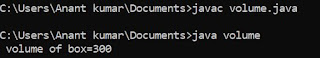## Friday, 23 November 2018

### Program to find maximum of three number using user define function in C++

A function can be define by the user as per the requirement of the user or programmer . To implement
a user define function , a programmer must contain the following three statement .

1. Function declaration or function prototype

2. Function call statement

3. Function definition

Here one new concept in C++ is INLINE function, Which we going to discuss in earlier post.

### Program of find maximum of three number using user define function in C++

#include <iostream.h>
#include<conio.h>
int max ( int , int , int ) ;
void main()
{
int a , b , c ;
clrscr () ;
cout<<"\n Enter value of a:" ;
cin>>a;
cout<<"\n Enter value of b:" ;
cin>>b;
cout<<"\n Enter value of c:" ;
cin>>c;
d = max ( a,b,c ) ;
cout<<" maximum number is"=<<"d<<"\n ;
getch () ;
}
int max ( int a , int b , int c )
{
if ( (a>b) && (a>c) )
return a ;
else if ( (b>a) && (b>c) )
return b ;
else
return c ;
}

This is program for finding maximum of three number in C++ using user define function. Here first of all we have to input three number from the user and write function call statement and print the value of maximum and outside the main function the function definition is written where the execution of program is takes place and output goes again to the function call statement where it is print on the output screen.

1.Please I cannot compile most of the codes here with codeblocks. Help me with another means of compiling

2.## Featured post

### Program to find Volume of given box in java

class volume { public static void main (String args []) { int l=5,b=6,h=10; int volume; volume=l*b*h; System.out.println (" vol...